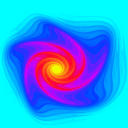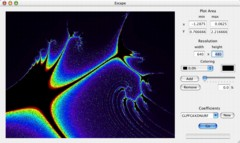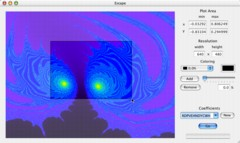Escape.app
 Escape.app is a Mac OS X application for generating escape time fractals. It supports both user input seeds and randomly generated seeds. You can zoom by either entering x and y coordinate ranges or by graphically selecting a zoom area on screen. By default, fractals are rendered in grayscale, but color gradients with arbitrarily many colors can also be specified.Escape time fractals are generated by feeding the x and y coordinates of a point into two equations. However, instead of plotting the outputs of these equations, the outputs are plugged back into the equations again and again. After the outputs finally reach some threshold distance from the center of the plot, we stop. But instead of plotting these final outputs, we plot the number of times we had to plug the outputs back into the original equations. So, each point in an escape time fractal is colored based on how many iterations it took for the point to "escape" past a certain threshold.Escape.app calculates escape times for arbitrary order-2 polynomials with coefficients ranging from -1.2 to 1.2 in increments of 0.1. So, the equations take the form: x_new = ax^2 + bx + cy^2 + dy + exy + f y_new = gx^2 + hx + iy^2 + jy + kxy + l
To refer to all the different possible equations which Escape.app uses, we name the fractals by assigning a letter to each possible coefficient value. A=-1.2, B=-1.1, C=-1.0 ... W=1.0, X=1.1, Y=1.2. So, the equations
x_new = (-1.0)x^2 + (-1.2)x + (-0.3)y^2 + (0.7)y + (-1.2)xy + (-1.1)
y_new = (1.0)x^2 + (0.7)x + (-1.1)y^2 + (0.1)y + (-0.9)xy + (1.1)

would be represented as CAJTABWTBNVX. This method for naming fractals of this type was proposed by Clifford Pickover, in his book Keys to Infinity. While using only equations of this form and only using 25 different possible values for each coefficient certainly limits the possible fractals, there are still 5.96 * 10^16 possibilities, and that's before you consider all of the possible colorings and zoom factors!

If you're interested in viewing some fractals generated by Escape.app, check out amiacoolfractalornot.org. There, you will be able to view and rate thousands of different fractals all of which were generated using Escape.app.